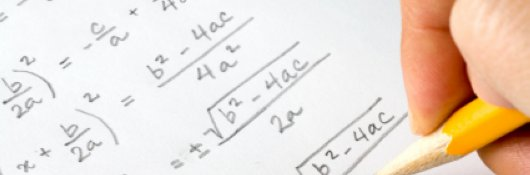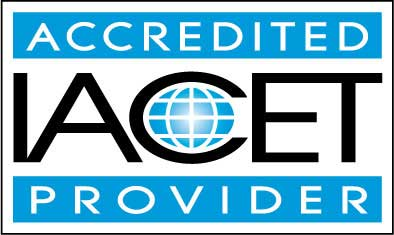# Course Syllabus## Course Description

Beginner to Intermediate Algebra is intended for students who need to gather a basic understanding of how to perform Algebra operations. This course will benefit current students and adult learners who need to know how to perform basic Algebra operations which involve simplifying, factoring, or solving equations in the form of inequalities, fractions, exponents and word problems.

This online course takes the student's knowledge of arithmetic and extends it by introducing the concepts of variables and functions. These new concepts allow students to solve a range of problems involving unknown quantities in areas such as geometry, probability and statistics, and various real-world scenarios.

Even from their childhood days, most people have at least a basic grasp of arithmetic: addition and subtraction of numbers, for instance. As we master these basic operations, we begin to search for a more systematic and rigorous understanding of the behavior of numbers (particularly, sets of numbers) and functional relationships. This search is where we make the step from arithmetic to algebra. Algebra is the systematic study of numbers (or, more generally, quantity) and their relationships at an abstract level. By studying algebra, you can acquire the ability to analyze the relationships between numbers generally, rather than simply between particular numbers. These abilities pave the way for understanding other areas of mathematics such as trigonometry and calculus.

• Completely Online
• Self-Paced
• 6 Months to Complete
• Start Anytime
• PC & Mac Compatible
• Android & iOS Friendly
• Accredited CEUs## Learning Outcomes

By successfully completing this course, students will be able to:
• Identify and define Algebra terms.
• Identify Real Numbers.
• Perform basic Algebraic Operations.
• Identify and create Algebra Functions.
• Identify and solve Linear Equations.
• Identify and solve Linear Inequalities.
• Identify and solve Algebra Word Problems.
• Identify and solve Polynomial Functions.
• Identify and solve Exponential and Logarithmic Functions.
• Demonstrate mastery of lesson content at levels of 70% or higher.

## Assessment Guide

Assessment Points
Introduction 1 points
Lesson 1 – Algebra Terms and Motivation 10 points
Lesson 2 – The Set of Real Numbers 10 points
Lesson 3 – Algebraic Operations 11 points
Lesson 3 Exercises 7 points
Lesson 4 - Functions 10 points
Lesson 4 Exercises 8 points
Lesson 5 – Linear Equations 10 points
Lesson 5 Exercises 9 points
Lesson 6 – Linear Inequalities 11 points
Lesson 6 Exercises 3 points
Lesson 7 – Systems of Linear Equations 10 points
Lesson 8 – Systems of Linear Inequalities 10 points
Lesson 9 – Solving Word Problems I 10 points
Lesson 9 Exercises 4 points
Lesson 10 – Polynomial Functions 10 points
Lesson 10 Exercises 8 points
Lesson 11 – Exponential and Logarithmic Functions 10 points
Lesson 12 – Polynomial Functions II 9 points
Lesson 12 Exercises 3 points
Lesson 13 – Application of Algebra to Geometry 9 points
Lesson 13 Exercises 4 points
Lesson 14 – Application of Algebra to Statistics and Probability 9 points
The Final Exam 47 points